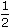# Aptitude - Boats and Streams

### Exercise :: Boats and Streams - General Questions

11.

A boatman goes 2 km against the current of the stream in 1 hour and goes 1 km along the current in 10 minutes. How long will it take to go 5 km in stationary water?

 A. 40 minutes B. 1 hour C. 1 hr 15 min D. 1 hr 30 min

Explanation:

 Rate downstream =1 x 60km/hr = 6 km/hr. 10

Rate upstream = 2 km/hr.

 Speed in still water = 1 (6 + 2) km/hr = 4 km/hr. 2Required time =5hrs = 1 1 hrs = 1 hr 15 min. 4 4

12.

A man can row three-quarters of a kilometre against the stream in 11minutes and down the stream in 7minutes. The speed (in km/hr) of the man in still water is:

 A. 2 B. 3 C. 4 D. 5

Explanation:

We can write three-quarters of a kilometre as 750 metres,

and 11minutes as 675 seconds.

 Rate upstream =750m/sec = 10 m/sec. 675 9

 Rate downstream =750m/sec = 5 m/sec. 450 3Rate in still water = 110 + 5m/sec 2 9 3

 = 25 m/sec 18

 =25 x 18km/hr 18 5

= 5 km/hr.

13.

Speed of a boat in standing water is 9 kmph and the speed of the stream is 1.5 kmph. A man rows to a place at a distance of 105 km and comes back to the starting point. The total time taken by him is:

 A. 16 hours B. 18 hours C. 20 hours D. 24 hours

Explanation:

Speed upstream = 7.5 kmph.

Speed downstream = 10.5 kmph.Total time taken =105 + 105hours = 24 hours. 7.5 10.5

14.

A man takes twice as long to row a distance against the stream as to row the same distance in favour of the stream. The ratio of the speed of the boat (in still water) and the stream is:

 A. 2 : 1 B. 3 : 1 C. 3 : 2 D. 4 : 3

Explanation:

Let man's rate upstream be x kmph.

Then, his rate downstream = 2x kmph.(Speed in still water) : (Speed of stream) =2x + x:2x - x2 2

 = 3x : x 2 2

= 3 : 1.

15.

A man rows to a place 48 km distant and come back in 14 hours. He finds that he can row 4 km with the stream in the same time as 3 km against the stream. The rate of the stream is:

 A. 1 km/hr B. 1.5 km/hr C. 2 km/hr D. 2.5 km/hr

Explanation:

Suppose he move 4 km downstream in x hours. Then,

 Speed downstream =4km/hr. x

 Speed upstream =3km/hr. x48 + 48 = 14 or x = 1 . (4/x) (3/x) 2

So, Speed downstream = 8 km/hr, Speed upstream = 6 km/hr.

 Rate of the stream = 1 (8 - 6) km/hr = 1 km/hr. 2